• Introduction
• What is in this manual
• What is Caspoc
• User interface
• Introduction
• Starting
• Simulation
• Editing
• Viewing and printing
• Getting Started
• Basic editing
• Simulation in the time domain
• Basic User Interface Topics
• Editing
• Simulation
• Viewing
• Library
• Reports
• Project management
• Circuit and Block Diagram Components
• Introduction
• Cscript and user defined functions
• Component parameters
• Modeling Topics
• Introduction
• Power Electronics
• Semiconductors
• Electrical Machines
• Electrical drives
• Power Systems
• Mechanical Systems
• Thermal Systems
• Magnetic Circuits
• Green Energy
• Coupling to FEM
• Experimenter
• Analog hardware description language
• Embedded C code Export
• Coupling to Spice
• Small Signal Analysis
• Matlab coupling
• Tips and tricks
• Appendices

## FEM Coupling Co-Simulation.

Co-simulation between FEM and the system simulation.

If all models in a system are such of complexity that they cannot be described by a set of parameters for an equivalent model, co-simulation is required. Also if the mathematical models of the components form one implicit set of equations that has to be solved 'in one go' instead of a sequential simulation, co-simulation is required.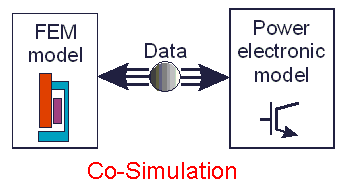A co-simulation is usually performed in the time domain and the results are waveforms from various observation points, like voltage, current speed, torque, power, pressure, etc.

Since all tools have to run at the same time, the tool that requires the most calculation times determines the total simulation time. For example, in a co-simulation of a transient FEM analysis of an electrical machine together with a circuit simulation of a power converter, the FEM analysis requires far more calculation time than the circuit simulation.

Example coupled simulation claw-pole generator and rectifier
Excerpt from:
Study on Novel Claw Pole Automobile Generator with Outer PM Rotor
Haijun Bai 1,2 , Fengge Zhang 1 , Hans Pert Gruenberger 3 , Eugen Nolle 3
1 Shenyang University of Technology, Shenyang, 110023,China
2 Shenyang Institute of Chemical Technology, Shenyang, 110142,China
3 Esslingen University of Applied Sciences, Esslingen, 73732,Germany
2007 Second IEEE Conference on Industrial Electronics and Applications

This section describes a permanent magnet claw pole generator (PMCPG) with outside rotor. The magnetic field distribution and induction EMF of the PMCPG are calculated by ANSYS, the power electronics are modeled in Caspoc. By means of two powerful simulation tools, the ANSYS and CASPOC software, the operation characteristic of the PMCPG with and without load is simulated on the basis of coupling of magnetic field and electric circuit. The simulation result shows that the PMCPG has a good operation performance and is suitable to be used in vehicles.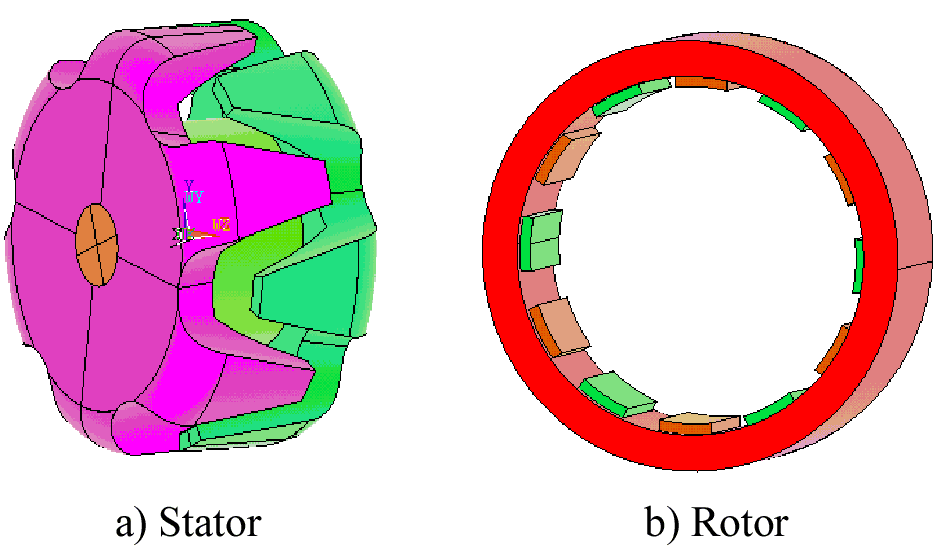A claw pole alternator has been used as the main source of electric energy in automobile for many years. The stator of this kind of claw pole generator is made up of insulated laminations and the stator�s slots carry a three-phase winding. The rotor consists of two claw pole wheels, the core with circumferential exciting coil and the shaft. Owing to its simplicity and low manufacturing cost, this generator is still used at present. However, its performance is hard to improve and the cost is hard to reduce further. Because the price of permanent magnet (PM) is lower and the performance of a new soft magnetic composite material (SMC) is very well, a novel permanent magnet claw pole machine with outside rotor will be described in this section. Its outside rotor is covered by PMs and stator of claw pole will be made of this kind of new material. This kind of material is magnetically isotropic due to its powdered nature and is suitable for construction of electric machine of complex structures. This kind of machine�s production cost will be much lower than the conventional claw pole machine and power electronics components which are used to rectify is fewer. In order to get the operation characteristic of this generator, the 3D electromagnetic analysis in ANSYS will be made in field calculation of electrical machine and meantime the electric-magnetic coupling calculation for PMCPG will be done by means of CASPOC software.

The PMCPG�s stator consists of two claw pole pieces mounted on a shaft with a single-phase concentrated winding between two pieces and it�s rotor comprises 12 surface-mounted PMs and a mild steel cylinder. The rotor is similar to a conventional PM machine. The stator structure is similar to the rotor of a conventional claw pole generator used at present.

When this machine operates as the generator, the rotor with PM pieces is rotated by other mechanism, a rotating magnetic field will be produced and forms magnetic loop by means of stator claws. In this situation, two sets of stator claws present alternately N or S Pole, so that a single phase AC EMF will be induced in the stator winding to output electricity energy.

In order to simulate the load characteristics of the claw pole generator, the simulation method of coupling ANSYS and CASPOC software has been applied.
ANSYS is used to analyze the magnetic field and calculate the electricmotive force, and its result is passed into the CASPOC software to calculate the value of stator winding current. The current obtained from CASPOC is received again by ANSYS, and the field analysis will start renewedly in ANSYS. The coupling calculation will keep running for the simulation time specified by the user. The flowchart of the ANSYS-CASPOC coupled simulation is shown below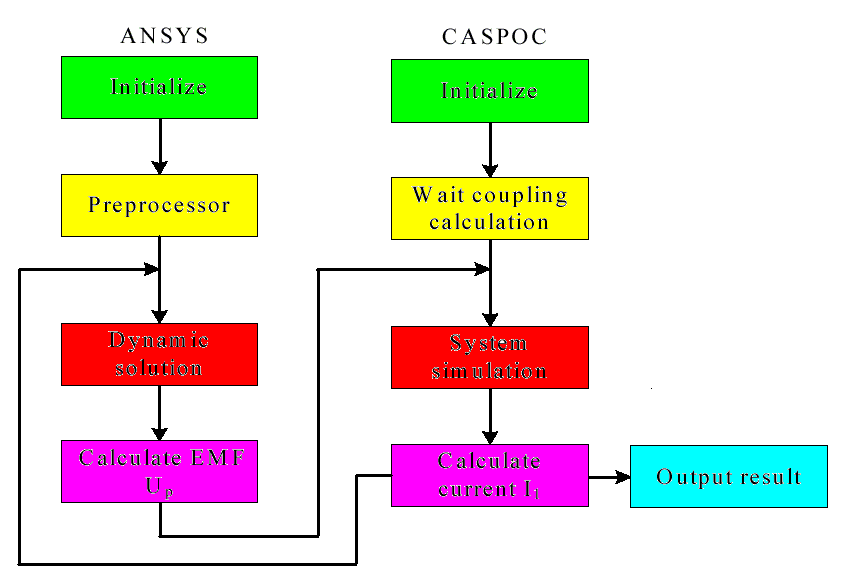A claw-pole generator supplies the storage battery and other electrical loads of vehicle by the four-diode bridge rectifier. The figure below shows the schematic diagram of CASPOC simulation model.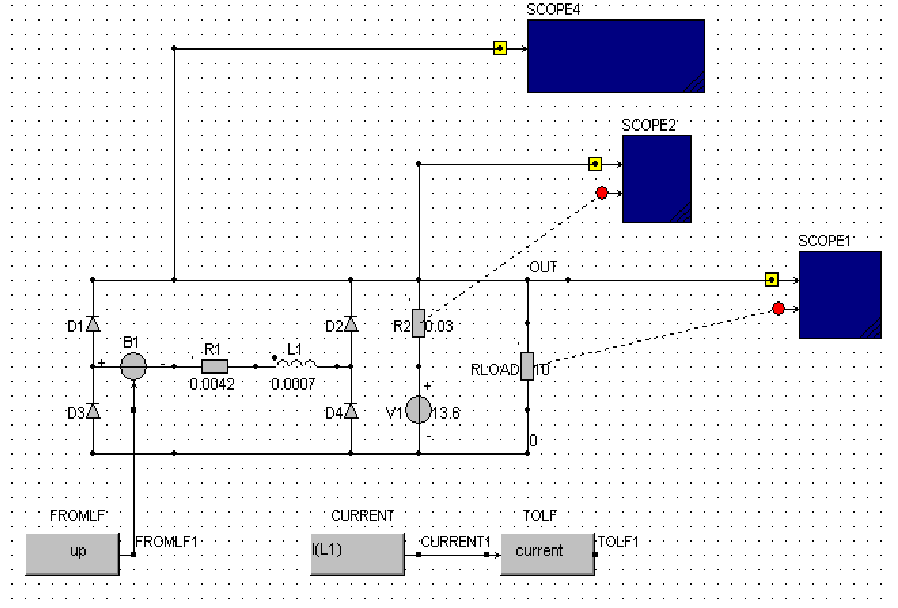In the figure above, the variable Up denotes the EMF and Il is winding current. CASPOC and ANSYS always wait for each other for the simulation result. Because the simulation of CASPOC is faster than ANSYS, it must wait for the data from ANSYS for some time by means of lock files. There is one point to be emphasized that time step of dynamic solution of ANSYS must be the same as that of CASPOC.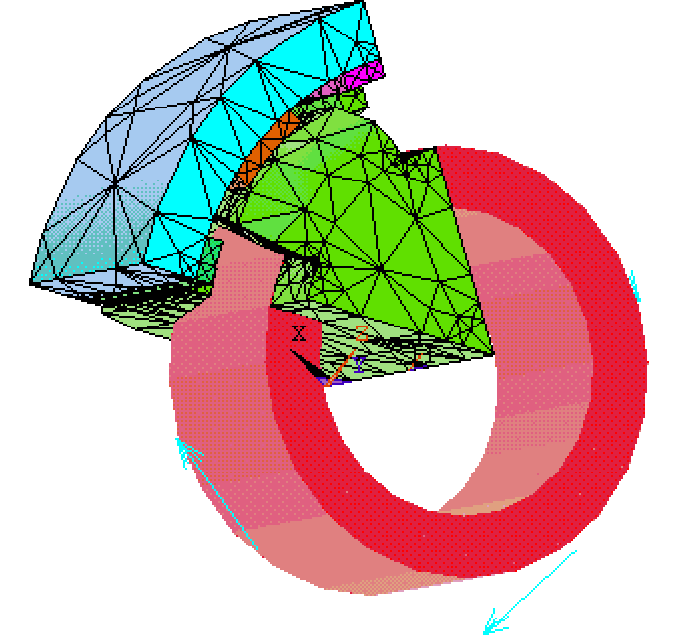The winding current is determined by calculating result of CASPOC and the EMF is induced under the composite magnetic fields and is transferred to CASPOC. Thus, the coupling computation will be made. The figure above shows the analytical mode of coupling calculation in ANSYS. After the outer rotor rotates 8 cycles, the output current and output voltage are stable.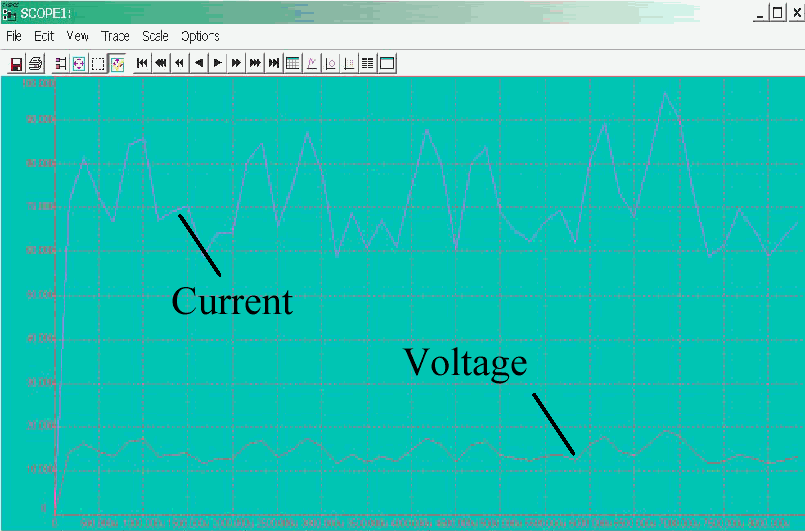The above figure shows the simulation result of the coupled ANSYS and CASPOC simulation. The upper curve is the winding current and its mean value is 73A. The lower curve is the output voltage and its mean value is 14V. Thus, this electrical machine may be used as the generator in automobile and it can satisfy the requirement of the electrical energy in vehicle.

As example of a co-simulation between Caspoc and FEM, the study of a novel permanent magnet claw pole generator with outside rotor is presented. Because the permanent magnets are used in outside rotor, the sliprings are not needed. The magnetic field distribution and induced back-EMF of the PMCPG have been calcualted by ANSYS. By means of the ANSYS and CASPOC simulation tools, the operation characteristic of the PMCPG with load is simulated on the basis of coupling of magnetic field and electric circuit. From the result of the analysis and calculation of the prototype machine, it can be concluded that the PMCPG has a good operation performance and is suitable to be used as a source of electrical energy in vehicles.